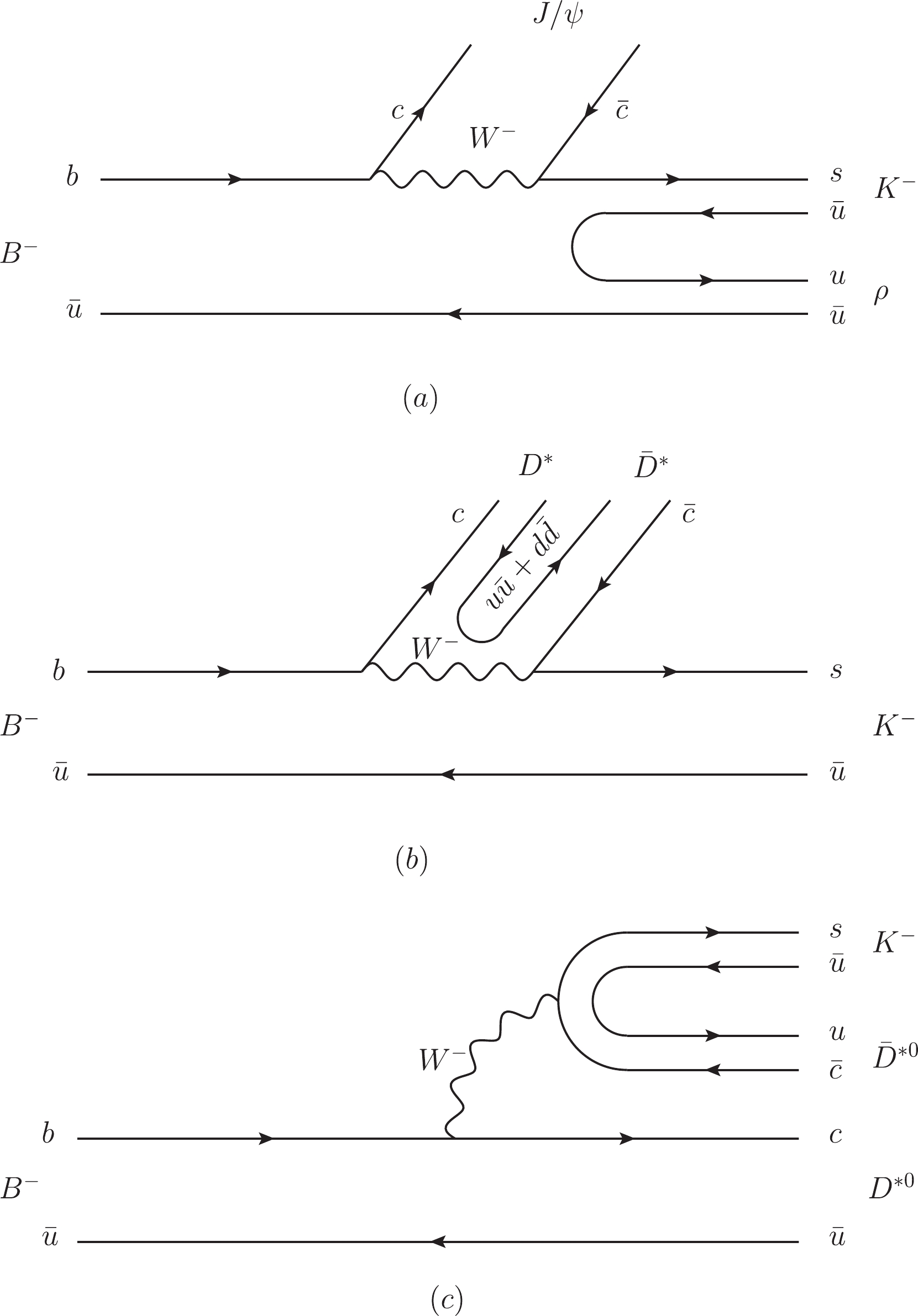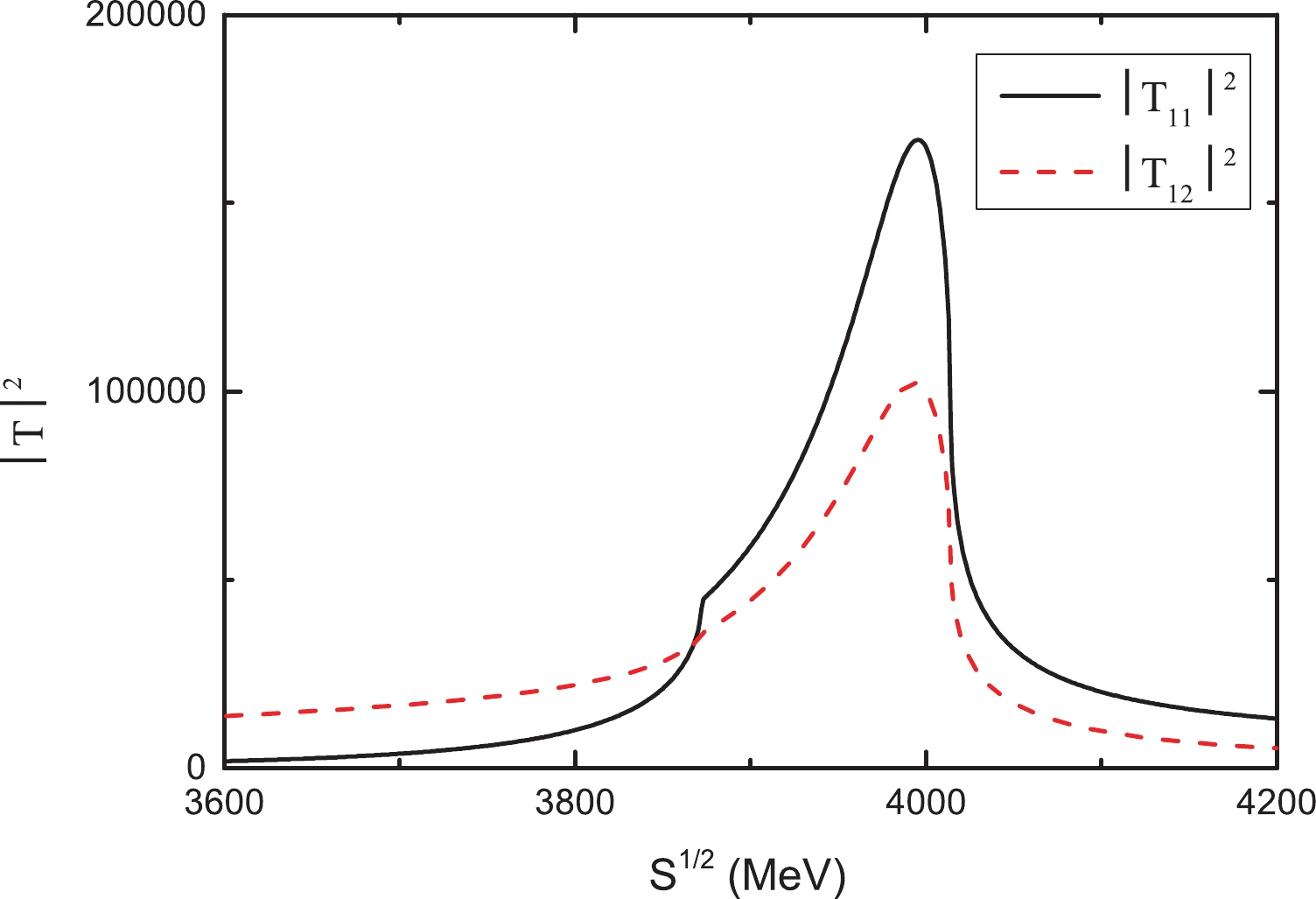# Search for the ${{{D^*}}\!{{\bar{D}^*}}}$molecular state ${{Z_c(4000)}}$in the reaction ${{B^{-}\! \rightarrow\! J/\psi \rho^0 K^{-}}}$• Based on the prediction of a $D^*\bar{D}^*$molecular state $Z_c(4000)$with isospin $I=1$in the coupled channel approach, we suggest the search for this state in the reaction $B^- \to J/\psi \rho^0 K^-$. By considering the final state interactions of $J/\psi \rho$and $D^{*0}\bar{D}^{*0}$and the contribution from the $K_1(1270)$resonance, we observed that the $J/\psi\rho$mass distribution shows a peak around 4000 MeV, which might be associated with the $D^*\bar{D}^*$molecular state $Z_c(4000)$. The search for $Z_c(4000)$in the reaction $B^- \to J/\psi \rho^0 K^-$is critical for understanding the internal structures of exotic hadrons. Our predictions can be tested by the Belle II and LHCb in future studies.
••Get Citation
Yang Zhang, En Wang, De-Min Li and Yu-Xiao Li. Search for the ${{{D^*}}\!{{\bar{D}^*}}}$molecular state ${{Z_c(4000)}}$in the reaction ${{B^{-}\! \rightarrow\! J/\psi \rho^0 K^{-}}}$[J]. Chinese Physics C. doi: 10.1088/1674-1137/44/9/093107
Yang Zhang, En Wang, De-Min Li and Yu-Xiao Li. Search for the ${{{D^*}}\!{{\bar{D}^*}}}$molecular state ${{Z_c(4000)}}$in the reaction ${{B^{-}\! \rightarrow\! J/\psi \rho^0 K^{-}}}$[J]. Chinese Physics C.Milestone
Received: 2020-04-16
Article Metric

Article Views(314)
PDF Downloads(26)
Cited by(0)
Policy on re-use
To reuse of Open Access content published by CPC, for content published under the terms of the Creative Commons Attribution 3.0 license (“CC CY”), the users don’t need to request permission to copy, distribute and display the final published version of the article and to create derivative works, subject to appropriate attribution.
###### 通讯作者: 陈斌, bchen63@163.com
• 1.

沈阳化工大学材料科学与工程学院 沈阳 110142

Title:
Email:

## Search for the ${{{D^*}}\!{{\bar{D}^*}}}$molecular state ${{Z_c(4000)}}$in the reaction ${{B^{-}\! \rightarrow\! J/\psi \rho^0 K^{-}}}$###### Corresponding author: En Wang, wangen@zzu.edu.cn
• School of Physics and Microelectronics, Zhengzhou University, Zhengzhou 450001, China

Abstract: Based on the prediction of a $D^*\bar{D}^*$molecular state $Z_c(4000)$with isospin $I=1$in the coupled channel approach, we suggest the search for this state in the reaction $B^- \to J/\psi \rho^0 K^-$. By considering the final state interactions of $J/\psi \rho$and $D^{*0}\bar{D}^{*0}$and the contribution from the $K_1(1270)$resonance, we observed that the $J/\psi\rho$mass distribution shows a peak around 4000 MeV, which might be associated with the $D^*\bar{D}^*$molecular state $Z_c(4000)$. The search for $Z_c(4000)$in the reaction $B^- \to J/\psi \rho^0 K^-$is critical for understanding the internal structures of exotic hadrons. Our predictions can be tested by the Belle II and LHCb in future studies.

### HTML2.   Formalism3.   Results
Reference (37)
PDF查看关注分享

Top

### 目录/DownLoad:  Full-Size Img  PowerPoint